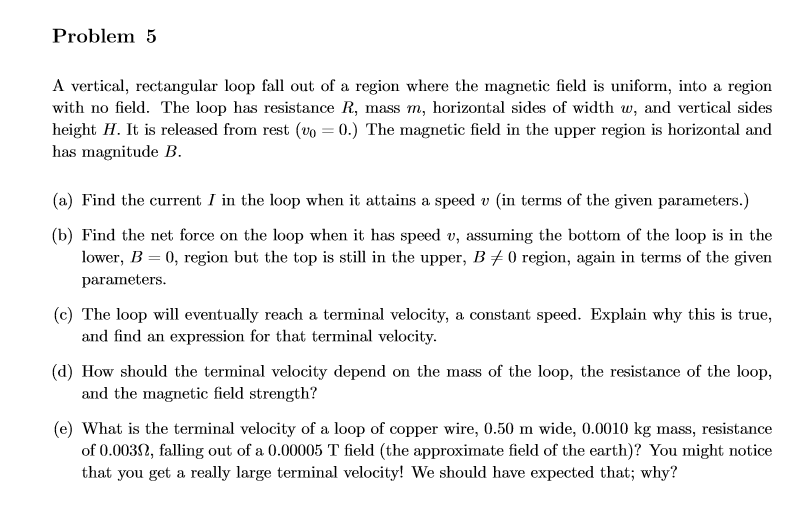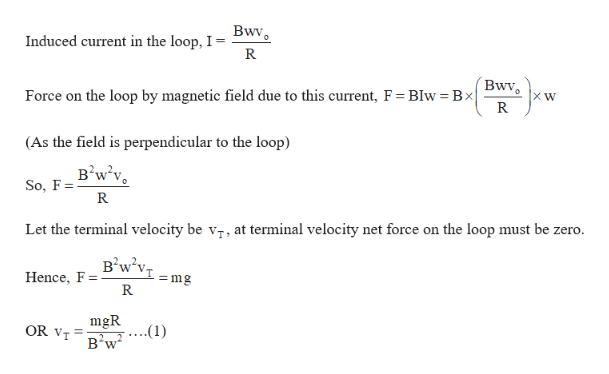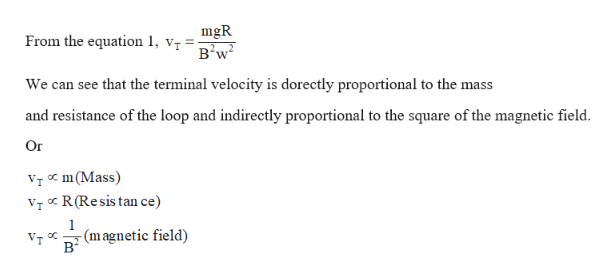# Problem 5A vertical, rectangular loop fall out of a region where the magnetic field is uniform, into a regionwith no field. The loop has resistance R, mass m, horizontal sides of width w, and vertical sidesheight H. It is released from rest (vo = 0.) The magnetic field in the upper region is horizontal andhas magnitude B(a) Find the current I in the loop when it attains a speed v (in terms of the given parameters.)(b) Find the net force on the loop when it has speed v, assuming the bottom of the loop is in thelower, B 0, region but the top is still in the upper, B /0 region, again in terms of the givenparameters(c) The loop will eventually reach a terminal velocity, a constant speed. Explain why this is true,and find an expression for that terminal velocity(d) How should the terminal velocity depend on the mass of the loop, the resistance of the loop,and the magnetic field strength?(e) What is the terminal velocity of a loop of copper wire, 0.50 m wide, 0.0010 kg mass, resistanceof 0.0032, falling out of a 0.00005 T field (the approximate field of the earth)? You might noticethat you get a really large terminal velocity! We should have expected that; why?

Question
36 views

Could you answer only D and E for me, thank you.help_outlineImage TranscriptioncloseProblem 5 A vertical, rectangular loop fall out of a region where the magnetic field is uniform, into a region with no field. The loop has resistance R, mass m, horizontal sides of width w, and vertical sides height H. It is released from rest (vo = 0.) The magnetic field in the upper region is horizontal and has magnitude B (a) Find the current I in the loop when it attains a speed v (in terms of the given parameters.) (b) Find the net force on the loop when it has speed v, assuming the bottom of the loop is in the lower, B 0, region but the top is still in the upper, B /0 region, again in terms of the given parameters (c) The loop will eventually reach a terminal velocity, a constant speed. Explain why this is true, and find an expression for that terminal velocity (d) How should the terminal velocity depend on the mass of the loop, the resistance of the loop, and the magnetic field strength? (e) What is the terminal velocity of a loop of copper wire, 0.50 m wide, 0.0010 kg mass, resistance of 0.0032, falling out of a 0.00005 T field (the approximate field of the earth)? You might notice that you get a really large terminal velocity! We should have expected that; why? fullscreen
check_circle

Step 1

Given:

Resistance of the loop = R

Mass of the loop = m

Horizontal length(Width) of the loop = w

Vertical length(length) of the loop = H

Initial velocity of the loop = 0 m/s

Magnetic field = B

Step 2

Calculating the terminal velocity of the loop:

Terminal velocity is the velocity when force of weight of loop becomes equal to the force on the loop by magnetic field due to induced current in the loop.So, when the net force on the loop becomes zero, the loop falls with a constant velocity, known as terminal velocity.help_outlineImage TranscriptioncloseBwv Induced current in the loop, I = R Bwv Force on the loop by magnetic field due to this current, F BIw Bx R (As the field is perpendicular to the loop) в'w'v. So, F R Let the terminal velocity be vr, at terminal velocity net force on the loop must be zero. в'w'v. mg Hence, F mgR ....(1) в'w OR vT fullscreen
Step 3

Determining how the terminal velocity depends on mas...help_outlineImage TranscriptionclosemgR From the equation 1, vr- We can see that the terminal velocity is dorectly proportional to the mass and resistance of the loop and indirectly proportional to the square of the magnetic field. Or vT om(Mass vT R(Resis tan ce) (magnetic field) fullscreen

### Want to see the full answer?

See Solution

#### Want to see this answer and more?

Solutions are written by subject experts who are available 24/7. Questions are typically answered within 1 hour.*

See Solution
*Response times may vary by subject and question.
Tagged in